0

# 十大经典排序算法（下）

🍓个人主页：bit..

🍒系列专栏：Linux(Ubuntu)入门必看 C语言刷题 数据结构与算法 HTML和CSS3

## 1.6 快速排序

### 1. 算法步骤

1. 从数列中挑出一个元素，称为 "基准"（pivot）;
2. 重新排序数列，所有元素比基准值小的摆放在基准前面，所有元素比基准值大的摆在基准的后面（相同的数可以到任一边）。在这个分区退出之后，该基准就处于数列的中间位置。这个称为分区（partition）操作；
3. 递归地（recursive）把小于基准值元素的子数列和大于基准值元素的子数列排序；

### 2. 动图演示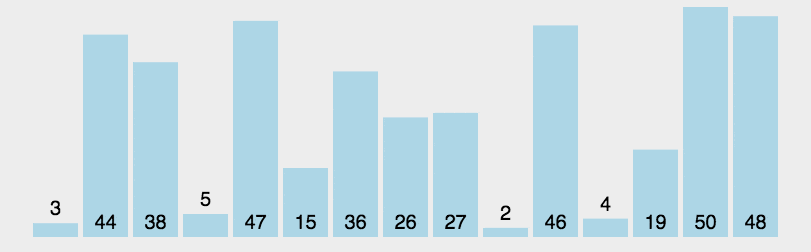### 3.代码实现

``````public class QuickSort implements IArraySort {

@Override
public int[] sort(int[] sourceArray) throws Exception {
// 对 arr 进行拷贝，不改变参数内容
int[] arr = Arrays.copyOf(sourceArray, sourceArray.length);

return quickSort(arr, 0, arr.length - 1);
}

private int[] quickSort(int[] arr, int left, int right) {
if (left < right) {
int partitionIndex = partition(arr, left, right);
quickSort(arr, left, partitionIndex - 1);
quickSort(arr, partitionIndex + 1, right);
}
return arr;
}

private int partition(int[] arr, int left, int right) {
// 设定基准值（pivot）
int pivot = left;
int index = pivot + 1;
for (int i = index; i <= right; i++) {
if (arr[i] < arr[pivot]) {
swap(arr, i, index);
index++;
}
}
swap(arr, pivot, index - 1);
return index - 1;
}

private void swap(int[] arr, int i, int j) {
int temp = arr[i];
arr[i] = arr[j];
arr[j] = temp;
}

}
``````

## 1.7 堆排序

1. 大顶堆：每个节点的值都大于或等于其子节点的值，在堆排序算法中用于升序排列；
2. 小顶堆：每个节点的值都小于或等于其子节点的值，在堆排序算法中用于降序排列；

### 1. 算法步骤

1. 创建一个堆 H[0……n-1]；
2. 把堆首（最大值）和堆尾互换；
3. 把堆的尺寸缩小 1，并调用 shift_down(0)，目的是把新的数组顶端数据调整到相应位置；
4. 重复步骤 2，直到堆的尺寸为 1。

### 2. 动图演示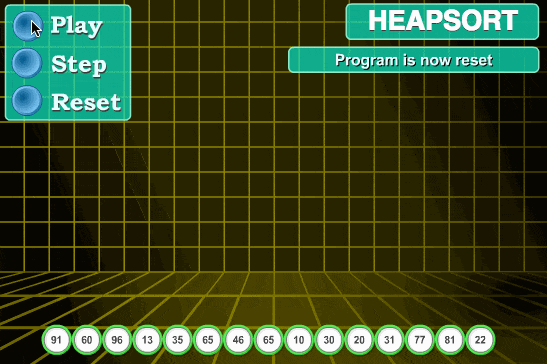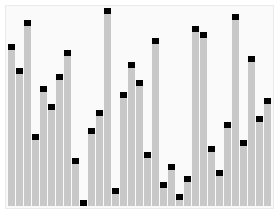### 3. 代码实现

``````public class HeapSort implements IArraySort {

@Override
public int[] sort(int[] sourceArray) throws Exception {
// 对 arr 进行拷贝，不改变参数内容
int[] arr = Arrays.copyOf(sourceArray, sourceArray.length);

int len = arr.length;

buildMaxHeap(arr, len);

for (int i = len - 1; i > 0; i--) {
swap(arr, 0, i);
len--;
heapify(arr, 0, len);
}
return arr;
}

private void buildMaxHeap(int[] arr, int len) {
for (int i = (int) Math.floor(len / 2); i >= 0; i--) {
heapify(arr, i, len);
}
}

private void heapify(int[] arr, int i, int len) {
int left = 2 * i + 1;
int right = 2 * i + 2;
int largest = i;

if (left < len && arr[left] > arr[largest]) {
largest = left;
}

if (right < len && arr[right] > arr[largest]) {
largest = right;
}

if (largest != i) {
swap(arr, i, largest);
heapify(arr, largest, len);
}
}

private void swap(int[] arr, int i, int j) {
int temp = arr[i];
arr[i] = arr[j];
arr[j] = temp;
}

}
``````

## 1.8 计数排序

### 1. 计数排序的特征

• （1）找出待排序的数组中最大和最小的元素
• （2）统计数组中每个值为i的元素出现的次数，存入数组C的第i项
• （3）对所有的计数累加（从C中的第一个元素开始，每一项和前一项相加）
• （4）反向填充目标数组：将每个元素i放在新数组的第C(i)项，每放一个元素就将C(i)减去1

### 2. 动图演示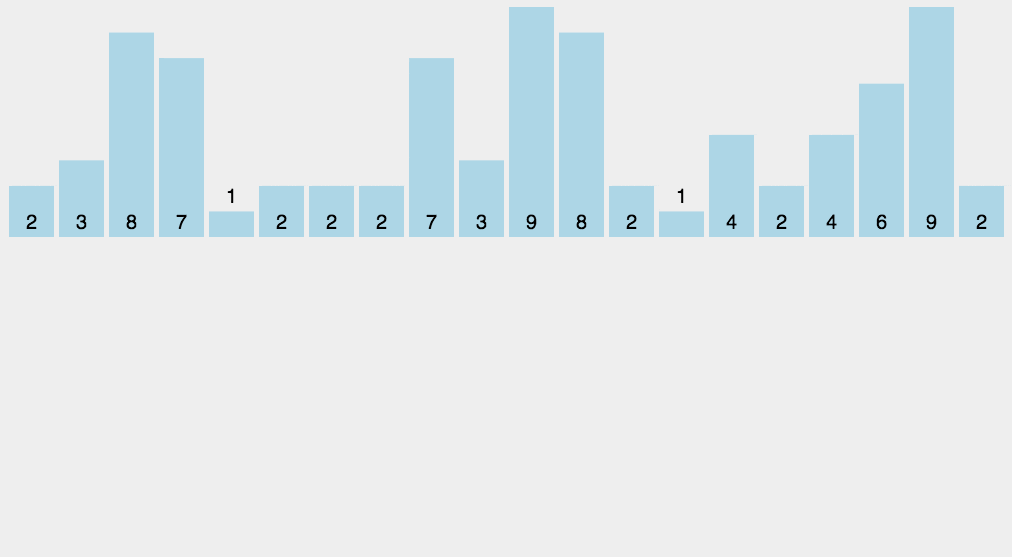### 3.代码实现

``````public class CountingSort implements IArraySort {

@Override
public int[] sort(int[] sourceArray) throws Exception {
// 对 arr 进行拷贝，不改变参数内容
int[] arr = Arrays.copyOf(sourceArray, sourceArray.length);

int maxValue = getMaxValue(arr);

return countingSort(arr, maxValue);
}

private int[] countingSort(int[] arr, int maxValue) {
int bucketLen = maxValue + 1;
int[] bucket = new int[bucketLen];

for (int value : arr) {
bucket[value]++;
}

int sortedIndex = 0;
for (int j = 0; j < bucketLen; j++) {
while (bucket[j] > 0) {
arr[sortedIndex++] = j;
bucket[j]--;
}
}
return arr;
}

private int getMaxValue(int[] arr) {
int maxValue = arr;
for (int value : arr) {
if (maxValue < value) {
maxValue = value;
}
}
return maxValue;
}

}
``````

## 1.9 桶排序

1. 在额外空间充足的情况下，尽量增大桶的数量
2. 使用的映射函数能够将输入的 N 个数据均匀的分配到 K 个桶中

### 3. 示意图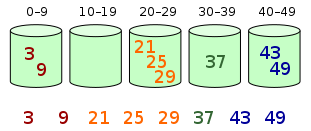### 3.代码实现

``````public class BucketSort implements IArraySort {

private static final InsertSort insertSort = new InsertSort();

@Override
public int[] sort(int[] sourceArray) throws Exception {
// 对 arr 进行拷贝，不改变参数内容
int[] arr = Arrays.copyOf(sourceArray, sourceArray.length);

return bucketSort(arr, 5);
}

private int[] bucketSort(int[] arr, int bucketSize) throws Exception {
if (arr.length == 0) {
return arr;
}

int minValue = arr;
int maxValue = arr;
for (int value : arr) {
if (value < minValue) {
minValue = value;
} else if (value > maxValue) {
maxValue = value;
}
}

int bucketCount = (int) Math.floor((maxValue - minValue) / bucketSize) + 1;
int[][] buckets = new int[bucketCount];

// 利用映射函数将数据分配到各个桶中
for (int i = 0; i < arr.length; i++) {
int index = (int) Math.floor((arr[i] - minValue) / bucketSize);
buckets[index] = arrAppend(buckets[index], arr[i]);
}

int arrIndex = 0;
for (int[] bucket : buckets) {
if (bucket.length <= 0) {
continue;
}
// 对每个桶进行排序，这里使用了插入排序
bucket = insertSort.sort(bucket);
for (int value : bucket) {
arr[arrIndex++] = value;
}
}

return arr;
}

/**
* 自动扩容，并保存数据
*
* @param arr
* @param value
*/
private int[] arrAppend(int[] arr, int value) {
arr = Arrays.copyOf(arr, arr.length + 1);
arr[arr.length - 1] = value;
return arr;
}

}
``````

## 1.10 基数排序

### 1. 基数排序 vs 计数排序 vs 桶排序

• 基数排序：根据键值的每位数字来分配桶；
• 计数排序：每个桶只存储单一键值；
• 桶排序：每个桶存储一定范围的数值；

### 2. LSD 基数排序动图演示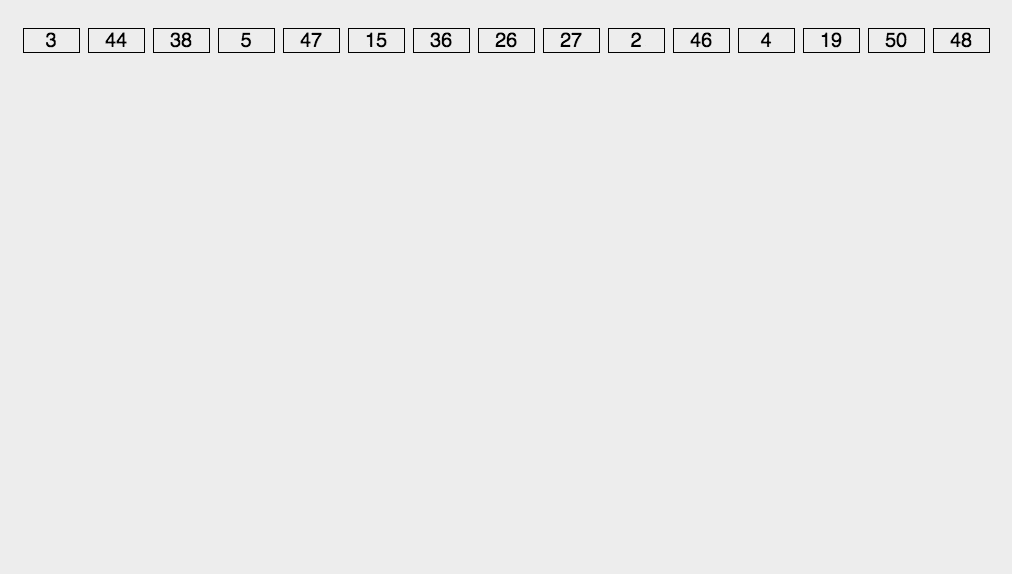### 3.代码实现

``````/**
* 基数排序
* 考虑负数的情况还可以参考： https://code.i-harness.com/zh-CN/q/e98fa9
*/
public class RadixSort implements IArraySort {

@Override
public int[] sort(int[] sourceArray) throws Exception {
// 对 arr 进行拷贝，不改变参数内容
int[] arr = Arrays.copyOf(sourceArray, sourceArray.length);

int maxDigit = getMaxDigit(arr);
}

/**
* 获取最高位数
*/
private int getMaxDigit(int[] arr) {
int maxValue = getMaxValue(arr);
return getNumLenght(maxValue);
}

private int getMaxValue(int[] arr) {
int maxValue = arr;
for (int value : arr) {
if (maxValue < value) {
maxValue = value;
}
}
return maxValue;
}

protected int getNumLenght(long num) {
if (num == 0) {
return 1;
}
int lenght = 0;
for (long temp = num; temp != 0; temp /= 10) {
lenght++;
}
return lenght;
}

private int[] radixSort(int[] arr, int maxDigit) {
int mod = 10;
int dev = 1;

for (int i = 0; i < maxDigit; i++, dev *= 10, mod *= 10) {
// 考虑负数的情况，这里扩展一倍队列数，其中 [0-9]对应负数，[10-19]对应正数 (bucket + 10)
int[][] counter = new int[mod * 2];

for (int j = 0; j < arr.length; j++) {
int bucket = ((arr[j] % mod) / dev) + mod;
counter[bucket] = arrayAppend(counter[bucket], arr[j]);
}

int pos = 0;
for (int[] bucket : counter) {
for (int value : bucket) {
arr[pos++] = value;
}
}
}

return arr;
}

/**
* 自动扩容，并保存数据
*
* @param arr
* @param value
*/
private int[] arrayAppend(int[] arr, int value) {
arr = Arrays.copyOf(arr, arr.length + 1);
arr[arr.length - 1] = value;
return arr;
}
}
``````

### “十大经典排序算法（下）”的评论:

##### 关于作者##### overfit同步小助手